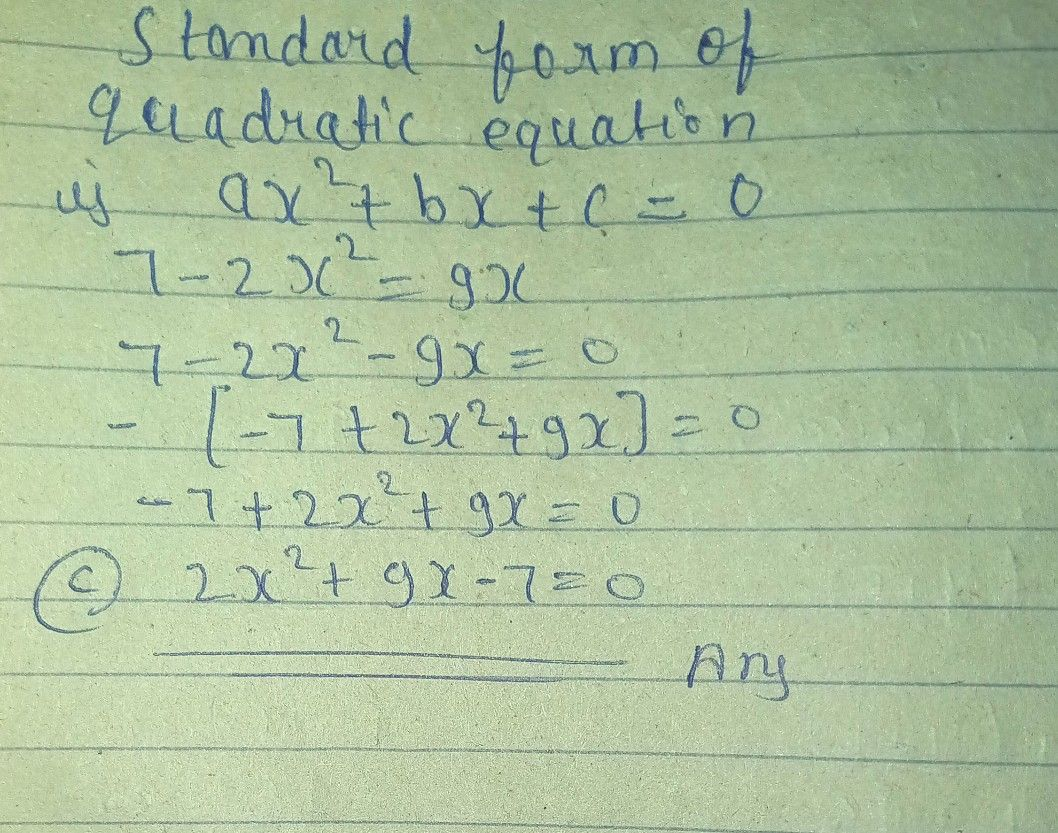Symbol
ProblemWhat is the standard form of the quadratic equation $7-2x^{2}=$ = $9x7$ a. $7-2x^{2}-9x=0$ c. $2x^{2}+9x$ $-7=0$ $b$ $-2x^{2}$ $-2x^{2}+7-9x=0$ d. $=$ $2x^{2}-9x-7=0$